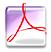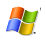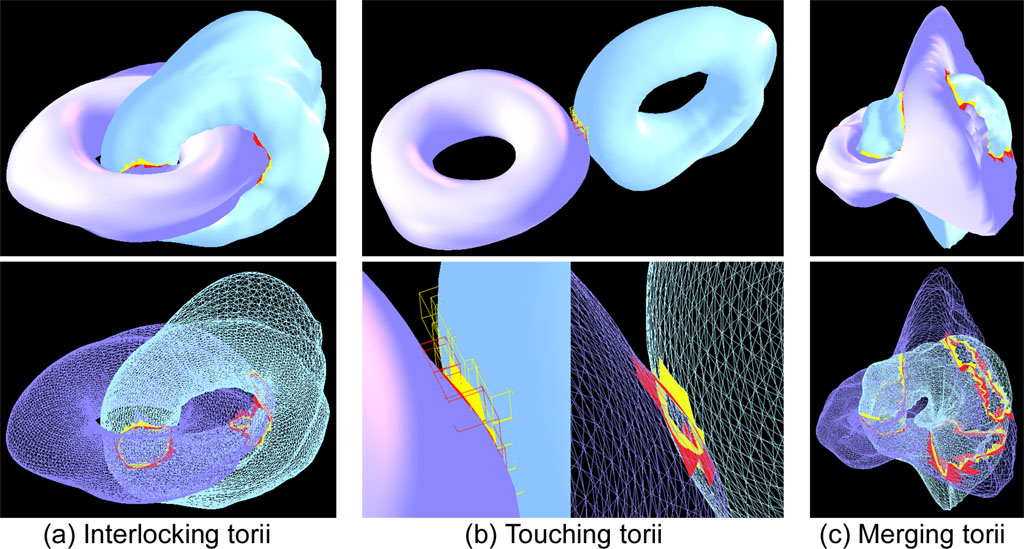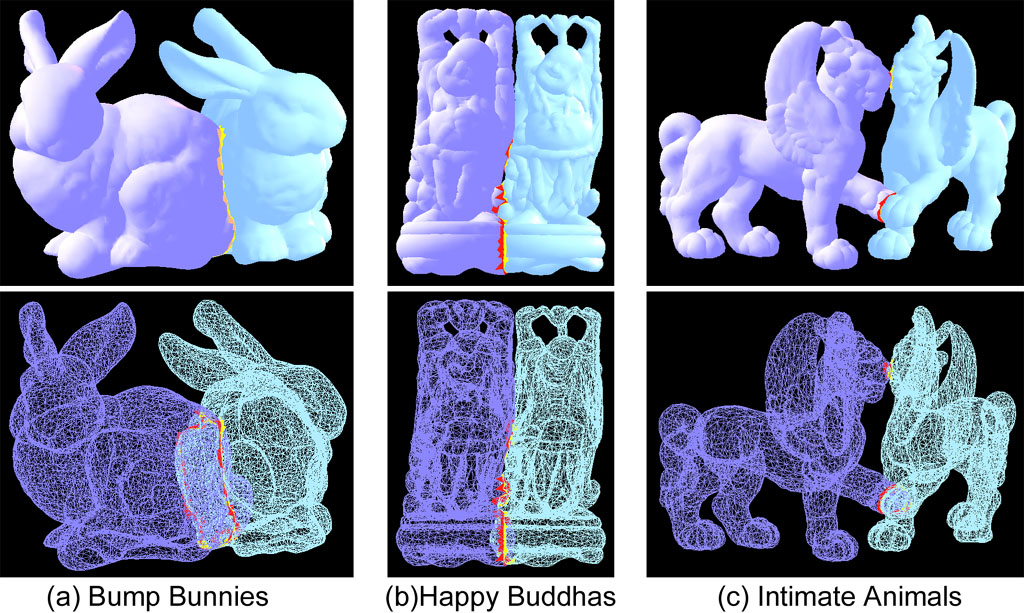Interactive Collision Detection for Deformable Models

Using Streaming AABBs

Abstract

 We present an interactive and accurate collision detection algorithm for deformable, polygonal objects based on the streaming computational model. Our algorithm can detect all possible pairwise primitive-level intersections between two severely deforming models at highly interactive rates.   In our streaming computational model, we consider a set of axis aligned bounding boxes (AABBs) that bound each of the given deformable objects as an input stream and perform massively-parallel pairwise, overlapping tests onto the incoming streams. As a result, we are able to prevent performance stalls in the streaming pipeline that can be caused by expensive indexing mechanism required by bounding volume hierarchy-based streaming algorithms. At run-time, as the underlying models deform over time, we employ a novel, streaming algorithm to update the geometric changes in the AABB streams. Moreover, in order to get only the computed result (i.e., collision results between AABBs) without reading back the entire output streams, we propose a streaming en/decoding strategy that can be performed in a hierarchical fashion. After determining overlapped AABBs, we perform a primitive-level (e.g., triangle) intersection checking on a serial computational model such as CPUs.   We implemented the entire pipeline of our algorithm using off-the-shelf graphics processors (GPUs), such as nVIDIA GeForce 6800, for streaming computations, and Intel Pentium 4 processors for serial computations. We benchmarked our algorithm with different models of varying complexities, ranging from 15K up to 50K triangles, under various deformation motions, and the timings were obtained as 30~100 FPS depending on the complexity of models and their relative configurations. Finally, we made extensive comparisons with a well-known GPU-based collision detection algorithm, CULLIDE and observed about three times performance improvement over the earlier approach.

Key Words

 Collision Detection, Deformable Models, Programmable Graphics Hardware, Streaming Computations, AABB

Full Textpdf (3.96MBytes)
 IEEE Transactions on Visualization and Computer Graphics 2007avi (35MBytes, with 320x240 resolution)avi (130MBytes, with 512x512 resolution)
 (These videos require the Indeo Codec 5.1. Before installation, read me)

Benchmarking ScenariosBenchmark Set I: Each torus consists of 15K triangles and the wave deformation is adopted to simulate the deformation. (a) Interlocking torii: our algorithm can exactly compute all the colliding triangle pairs at update rate of 60-80 FPS. (b) Touching torii: our algorithm can exactly compute all the colliding triangle pairs at update rate of 90-100 FPS. (c) Merging torii: our algorithm can exactly compute all the colliding triangle pairs at update rate of 25-30 FPS.Benchmark Set II: The pulsating deformation is adopted to simulate the deformation. (a) Bump Bunnies: Each bunny consists of 15K triangles. Our algorithm can exactly compute all the colliding triangle pairs at update rate of 50-60 FPS. (b) Happy Buddhas: Each Buddha consists of 20K triangles. Our algorithm can exactly compute all the colliding triangle pairs at update rate of 25-40 FPS. (c) Intimate Animals: Each animal consists of 50K triangles. Our algorithm can exactly compute all the colliding triangle pairs at update rate of 20-35 FPS.

 Copyright 2005 Computer Graphics Laboratory Dept of Computer Science & Engineering Ewha Womans University, Seoul, Korea Last update: December 24, 2005